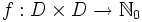# Conjugacy class-cum-order statistics of a finite group

## Definition

The conjugacy class-cum-order statistics of a finite group is a bunch of statistics that answers the question: how many conjugacy classes are there of a given size, and whose elements have a given order?

For a finite group$G$ with order$n$, let$D$ be the set of divisors of$n$. The conjugacy class-cum-order statistics is a function$f:D \times D \to \mathbb{N}_0$, with$f(d_1,d_2)$ equals the number of conjugacy classes of$G$ with size$d_1$ and whose elements have order$d_2$.

Note that since order of element divides order of group and size of conjugacy class divides order of group, it is valid to restrict both inputs to$f$ to divisors of$n$.

## Relation with subgroup-defining functions

Subgroup-defining function Information revealed by conjugacy class-cum-order statistics
Center Determined uniquely up to isomorphism. This is because it is precisely the set of elements with conjugacy class of size one, and it is abelian, and finite abelian groups with the same order statistics are isomorphic